## Topic: Geometry & Shapes

Free Printable Worksheets on Geometry and Shapes

## Geomety: 2D Shapes – Count the Sides (Hexagon)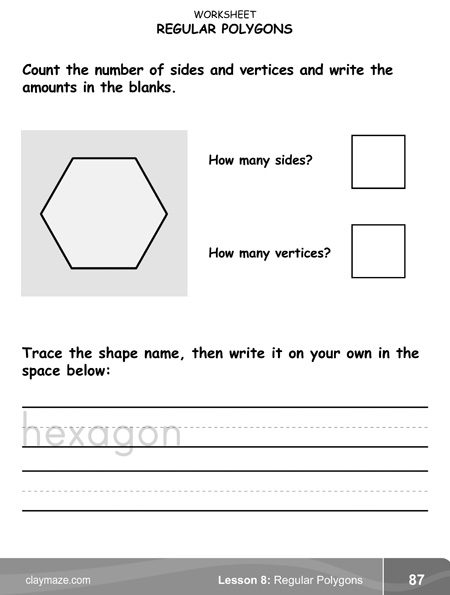This shapes worksheet is from the book, “Mastering the Math Milestone“. This worksheet has the child count sides and vertices, write that number and then trace and write the...

## Shapes and Dimensions: Rectangles and SquaresThis worksheet is from the “Mastering the Math Milestone” book from the chapter on shapes and dimensions. This worksheet is practice in understanding the relationship between width...

## Match the Numbers and Colors (Level1)Match the numbers and the colors by drawing lines from the center numbers to the correct number names and color names....

## Match the Numbers and Colors (Level 2)Draw lines to match the food names to the numbers that are the same color as the food and then match those numbers to the correct number names....

## Match the Shapes and Colors (Level 1, Page 1)Match the colors and shapes by drawing lines from the center shapes to the correct color names and shape names....

## Match the Shapes and Colors (Level 1, Page 2)Draw lines to match the shapes to the correct color names and shape names....

## Match the Shapes and Colors (Level 2, Page 1)Match the shapes to the correct shape names and color names....

## Match the Shapes and Colors (Level 2, Page 2)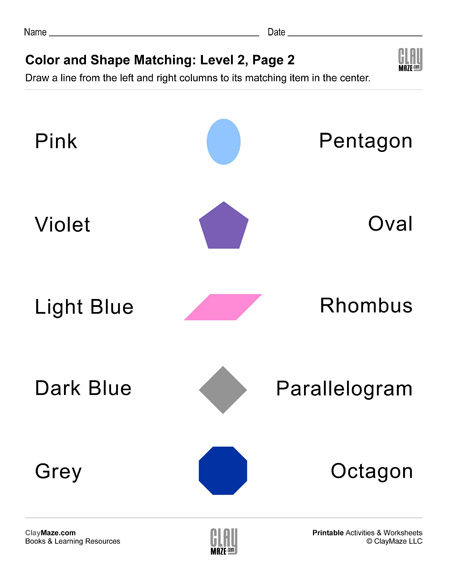Draw lines from the center shapes to the correct shape names and colors....

## Geometry: Find the Perimeter (Set 1)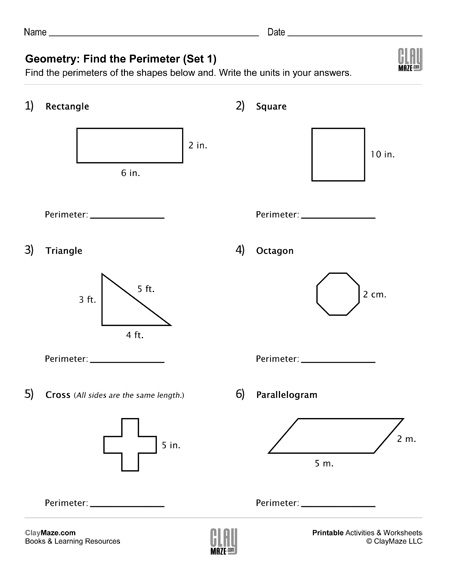Download this geometry worksheet on perimeters. Have the student find the perimeters of each of the shapes shown. This sheet features a rectangle, a square, a triangle, an octagon, a cross and a...

## Geometry: Find the Perimeter (Set 2)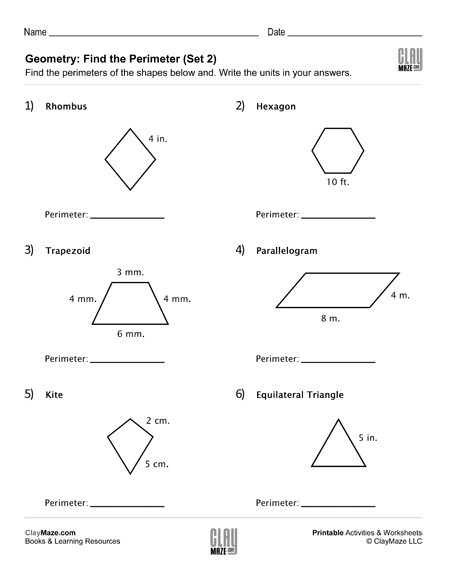Use this free worksheet for practice finding perimeters. The student is asked to find the perimeter of each of the shapes given. This worksheet features a rhombus, a hexagon, a trapezoid, a...

## Geometry: Find the Missing Angle in the Triangle (Set 1)This worksheet is for finding a missing angle in a triangle and is based on the student knowing that there are 180 degrees in a triangle. It also assumes the student knows the different types of...

## Geometry: Find the Missing Angle in the Triangle (Set 2)Download this worksheet on finding the missing angle in a triangle. This worksheet assume the student is familiar with right, isosceles and scalene triangles and knows that the angles in a...

## Geometry: Find the Missing Angle in the Triangle (Set 3)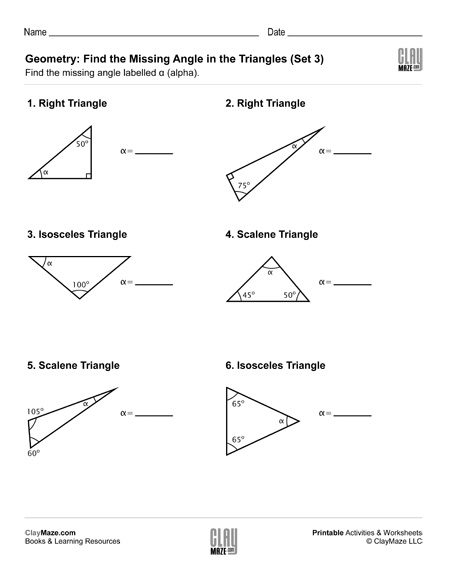Geometry worksheet on triangles and angles. The student is asked to find the unknown angle using their knowledge of triangles....

## Geometry: Find the Missing Angle in the Triangle (Set 4)In this worksheet the student is asked to find the missing angle in the triangles shown. This sheet is for students that are familiar with right, scalene and isosceles triangles....

## Complete the Sequences Worksheet Activity – Set 1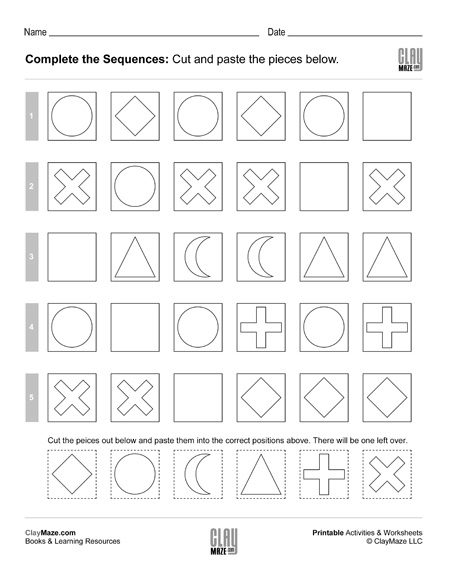Cut out the shapes and paste them into the correct position in the sequences....